# Properties of matter Questions and Answers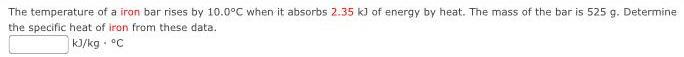Physics
Properties of matter
The temperature of a iron bar rises by 10.0°C when it absorbs 2.35 kJ of energy by heat. The mass of the bar is 525 g. Determine the specific heat of iron from these data. kJ/kg. °C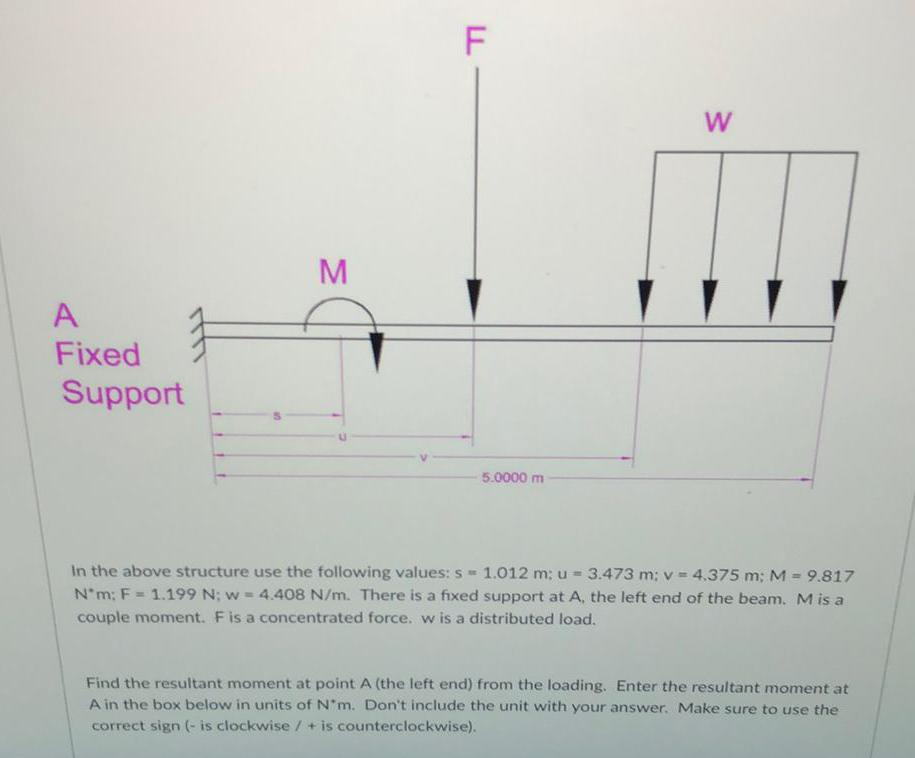Physics
Properties of matter
In the above structure use the following values: s= 1.012 m; u = 3.473 m; v = 4.375 m; M = 9.817 N*m; F = 1.199 N; w= 4.408 N/m. There is a fixed support at A, the left end of the beam. M is a couple moment. F is a concentrated force. w is a distributed load. Find the resultant moment at point A (the left end) from the loading. Enter the resultant moment at A in the box below in units of N*m. Don't include the unit with your answer. Make sure to use the correct sign (- is clockwise / + is counterclockwise).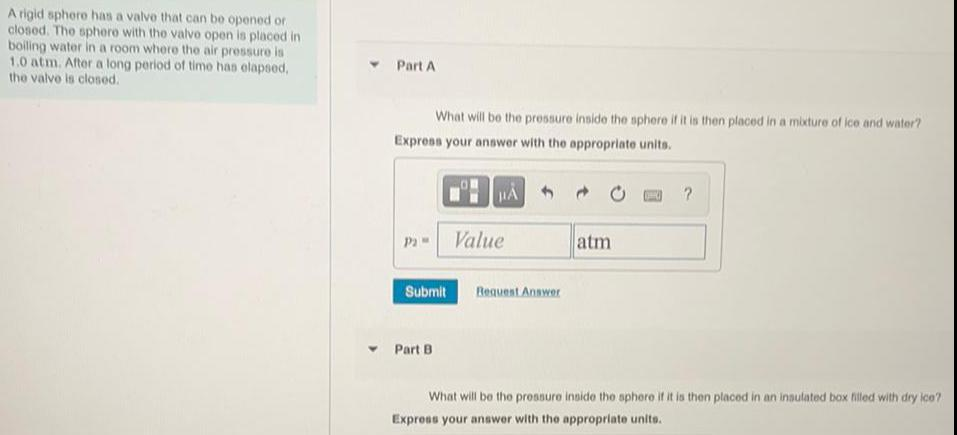Physics
Properties of matter
A rigid sphere has a valve that can be opened or closed. The sphere with the valve open is placed in boiling water in a room where the air pressure is 1.0 atm. After a long period of time has elapsed, the valve is closed. Part A What will be the pressure inside the sphere if it is then placed in a mixture of ice and water? Express your answer with the appropriate units. p2 Part B What will be the pressure inside the sphere if it is then placed in an insulated box filled with dry ice? Express your answer with the appropriate units.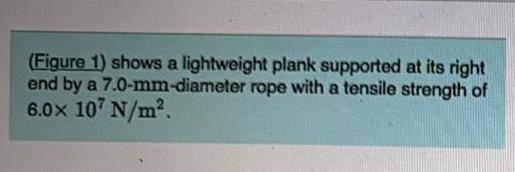Physics
Properties of matter
(Figure 1) shows a lightweight plank supported at its right end by a 7.0-mm-diameter rope with a tensile strength of 6.0x 10^7 N/m².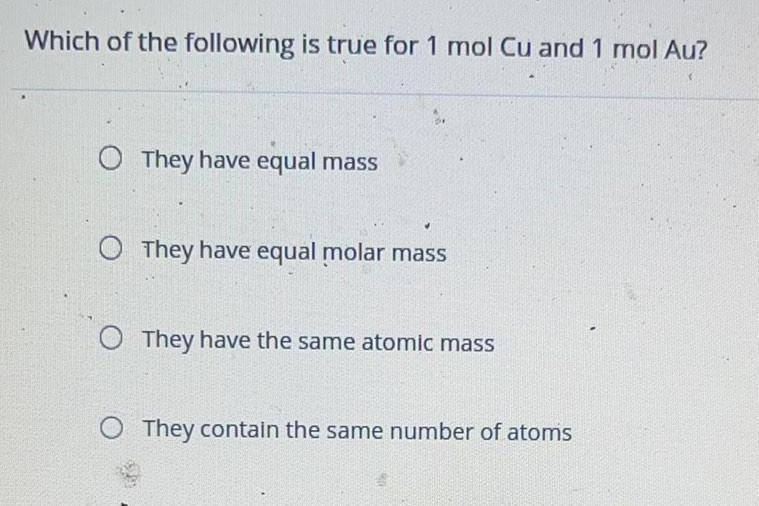Physics
Properties of matter
Which of the following is true for 1 mol Cu and 1 mol Au? They have equal mass They have equal molar mass They have the same atomic mass They contain the same number of atoms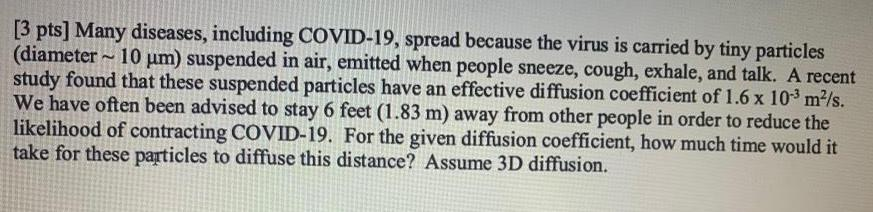Physics
Properties of matter
Many diseases, including COVID-19, spread because the virus is carried by tiny particles (diameter 10 µm) suspended in air, emitted when people sneeze, cough, exhale, and talk. A recent study found that these suspended particles have an effective diffusion coefficient of 1.6 x 10-³ m²/s. We have often been advised to stay 6 feet (1.83 m) away from other people in order to reduce the likelihood of contracting COVID-19. For the given diffusion coefficient, how much time would it take for these particles to diffuse this distance? Assume 3D diffusion.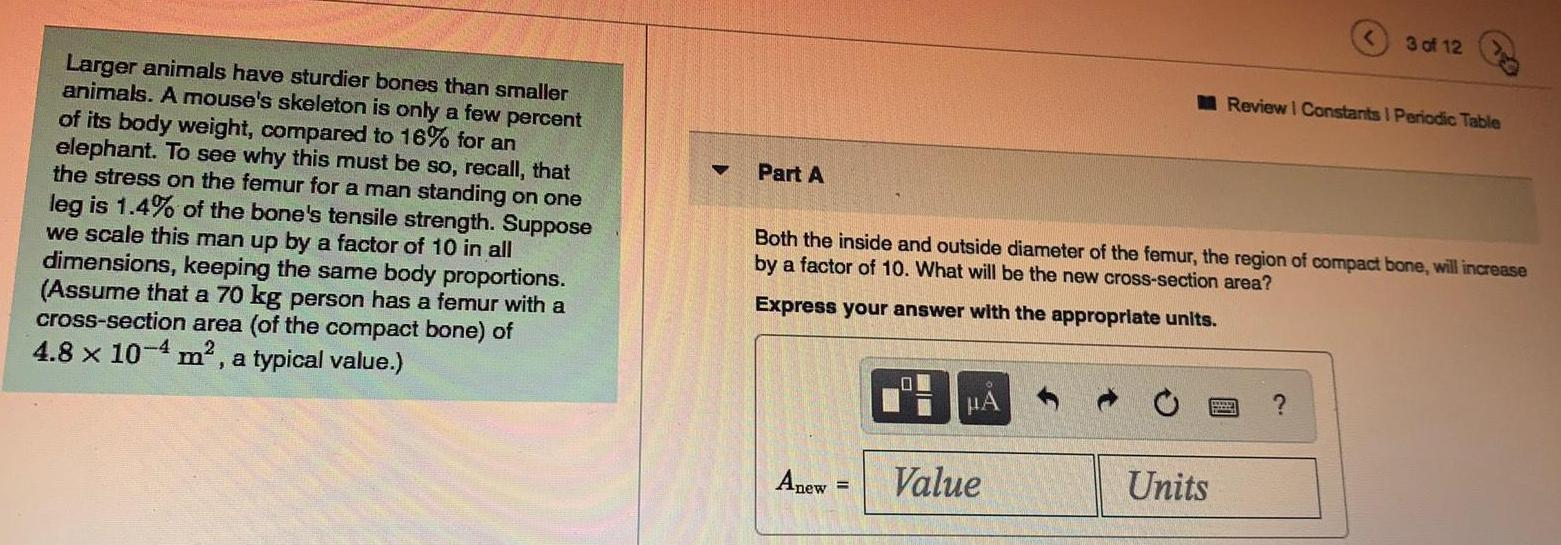Physics
Properties of matter
Larger animals have sturdier bones than smaller animals. A mouse's skeleton is only a few percent of its body weight, compared to 16% for an elephant. To see why this must be so, recall, that the stress on the femur for a man standing on one leg is 1.4% of the bone's tensile strength. Suppose we scale this man up by a factor of 10 in all dimensions, keeping the same body proportions. (Assume that a 70 kg person has a femur with a cross-section area (of the compact bone) of 4.8 x 10^-4 m², a typical value.) Both the inside and outside diameter of the femur, the region of compact bone, will increase by a factor of 10. What will be the new cross-section area?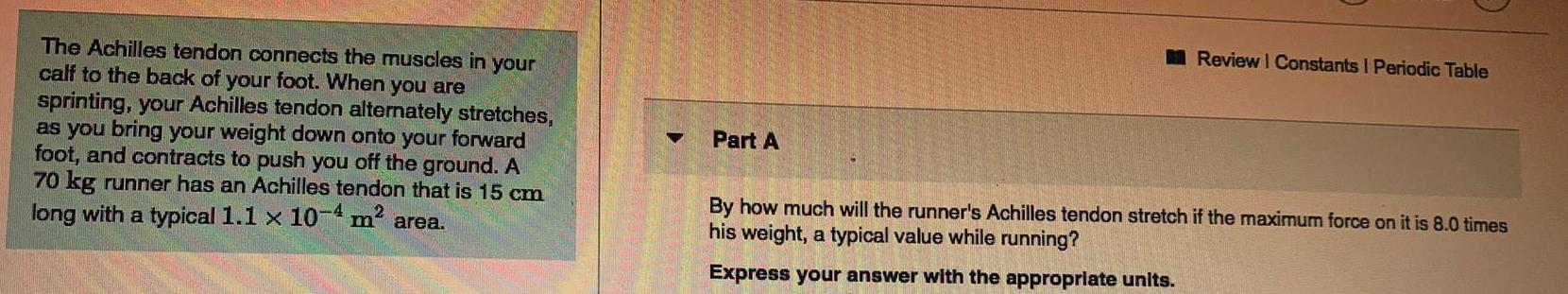Physics
Properties of matter
The Achilles tendon connects the muscles in your calf to the back of your foot. When you are sprinting, your Achilles tendon alternately stretches, as you bring your weight down onto your forward foot, and contracts to push you off the ground. A 70 kg runner has an Achilles tendon that is 15 cm long with a typical 1.1 x 10-4 m² area. By how much will the runner's Achilles tendon stretch if the maximum force on it is 8.0 times his weight, a typical value while running? Express your answer with the appropriate units.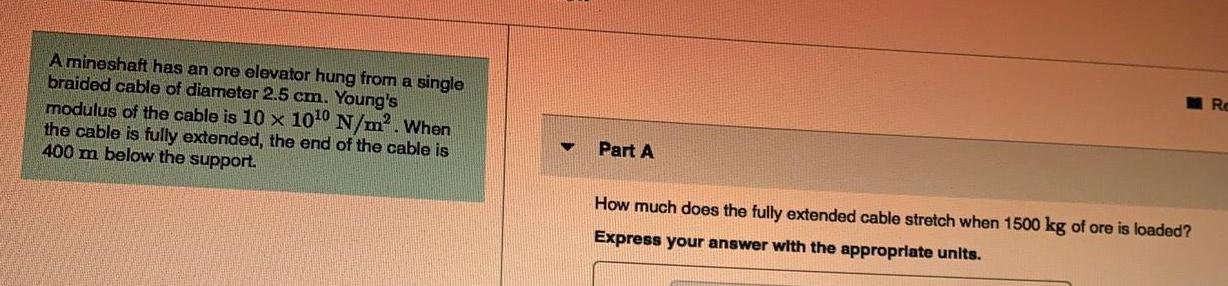Physics
Properties of matter
A mineshaft has an ore elevator hung from a single braided cable of diameter 2.5 cm. Young's modulus of the cable is 10 x 10^10 N/m². When the cable is fully extended, the end of the cable is 400 m below the support. Part A How much does the fully extended cable stretch when 1500 kg of ore is loaded?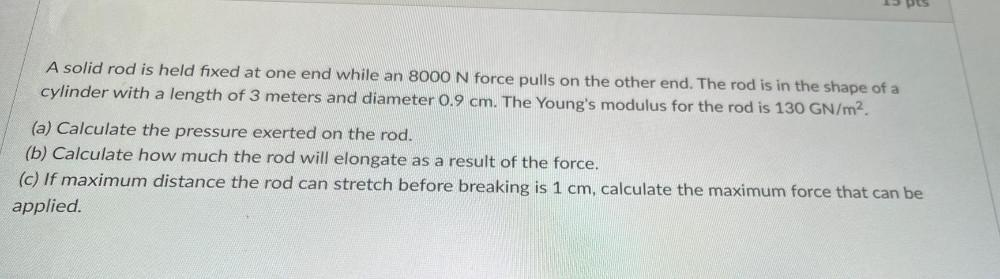Physics
Properties of matter
A solid rod is held fixed at one end while an 8000 N force pulls on the other end. The rod is in the shape of a cylinder with a length of 3 meters and diameter 0.9 cm. The Young's modulus for the rod is 130 GN/m². (a) Calculate the pressure exerted on the rod. (b) Calculate how much the rod will elongate as a result of the force. (c) If maximum distance the rod can stretch before breaking is 1 cm, calculate the maximum force that can be applied.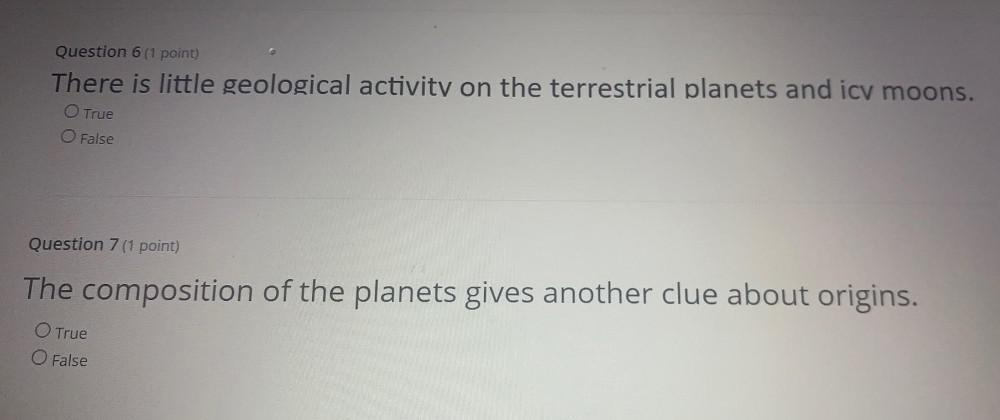Physics
Properties of matter
There is little geological activity on the terrestrial planets and icv moons. True False The composition of the planets gives another clue about origins. True False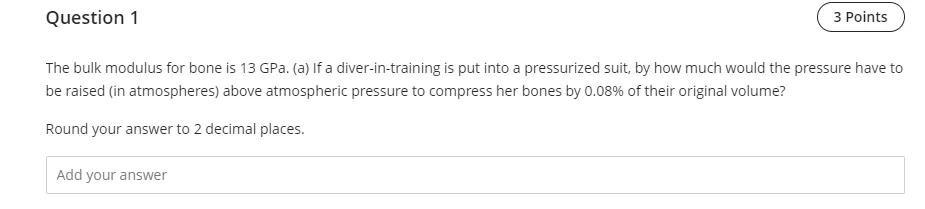Physics
Properties of matter
The bulk modulus for bone is 13 GPa. (a) If a diver-in-training is put into a pressurized suit, by how much would the pressure have to be raised (in atmospheres) above atmospheric pressure to compress her bones by 0.08% of their original volume?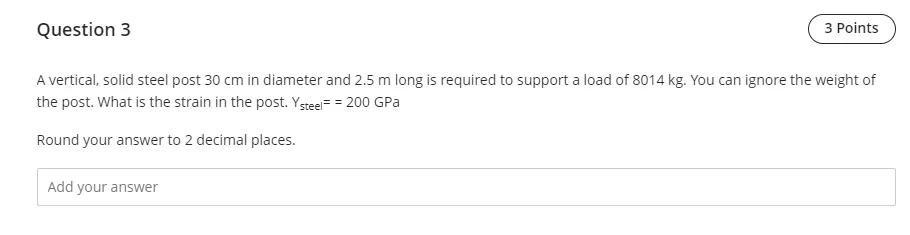Physics
Properties of matter
A vertical, solid steel post 30 cm in diameter and 2.5 m long is required to support a load of 8014 kg. You can ignore the weight of the post. What is the strain in the post. Ysteel == 200 GPa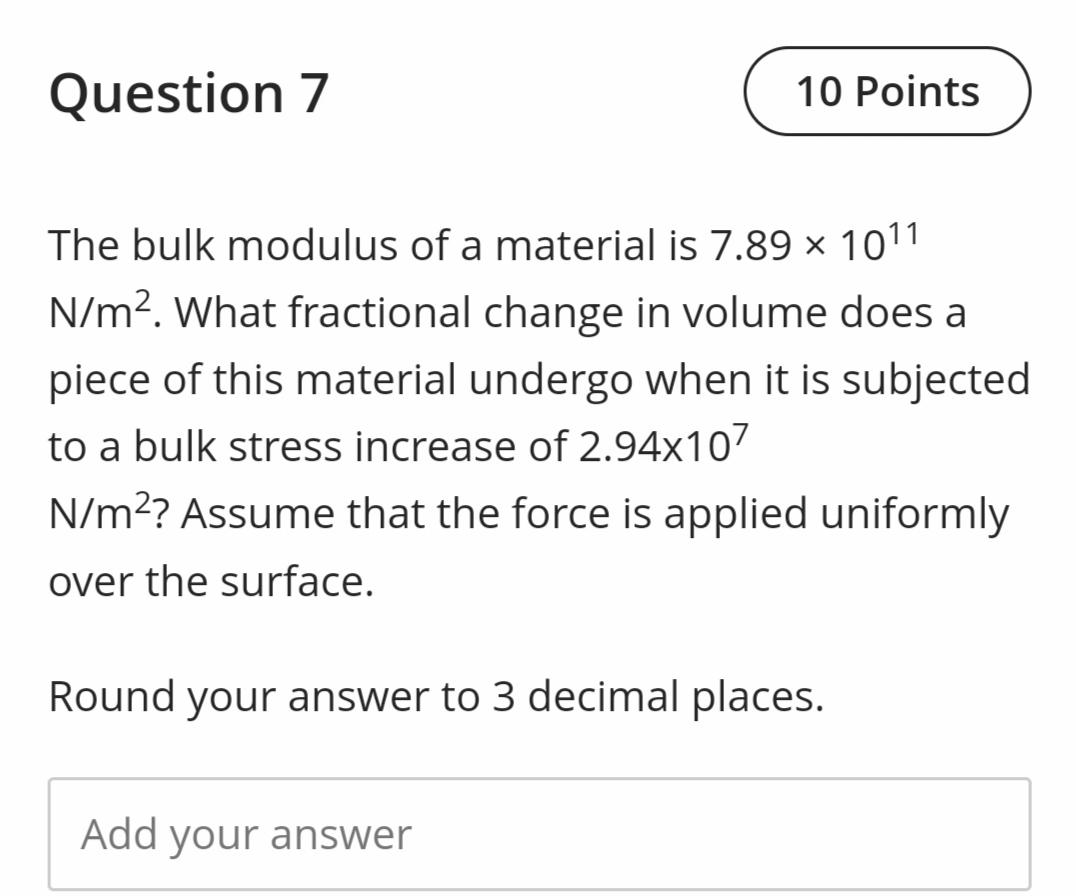Physics
Properties of matter
The bulk modulus of a material is 7.89 x 10¹¹ N/m². What fractional change in volume does a piece of this material undergo when it is subjected to a bulk stress increase of 2.94x10⁷ N/m²? Assume that the force is applied uniformly over the surface. Round your answer to 3 decimal places.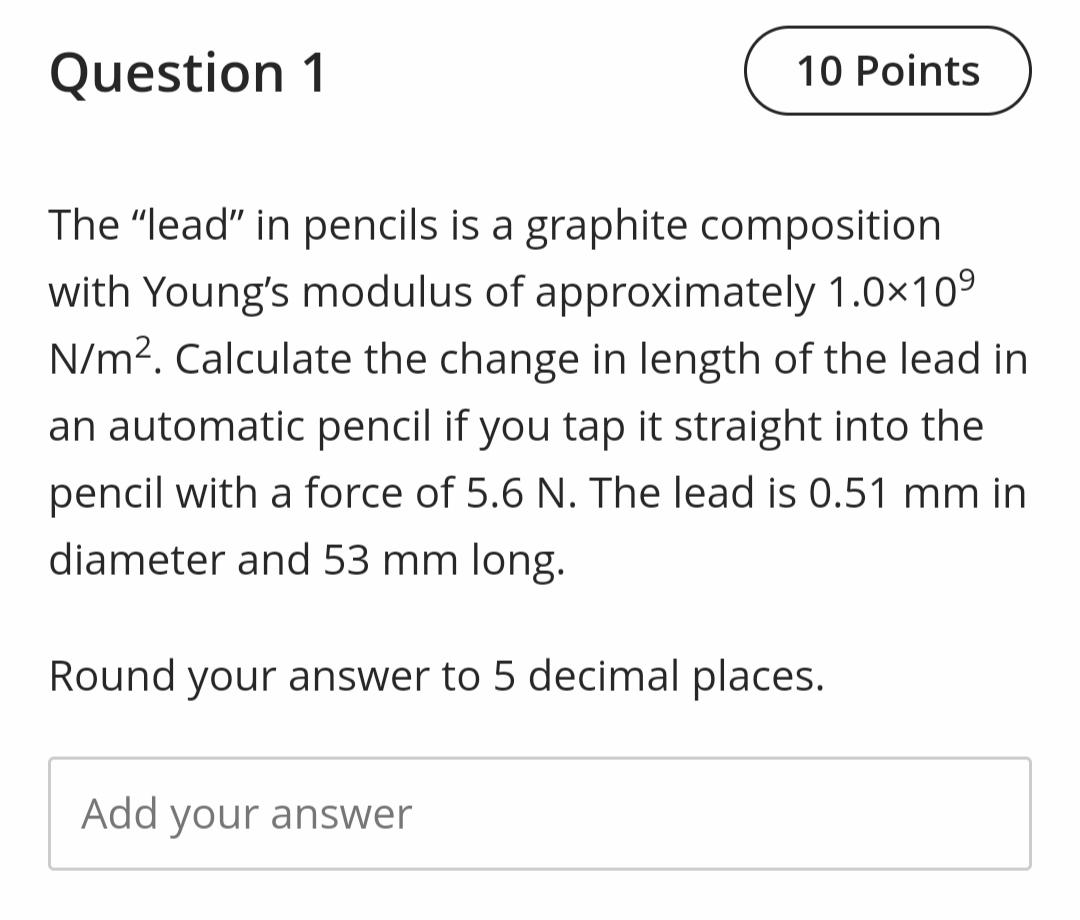Physics
Properties of matter
The “lead” in pencils is a graphite composition with Young's modulus of approximately 1.0x10⁹ N/m². Calculate the change in length of the lead in an automatic pencil if you tap it straight into the pencil with a force of 5.6 N. The lead is 0.51 mm in diameter and 53 mm long. Round your answer to 5 decimal places.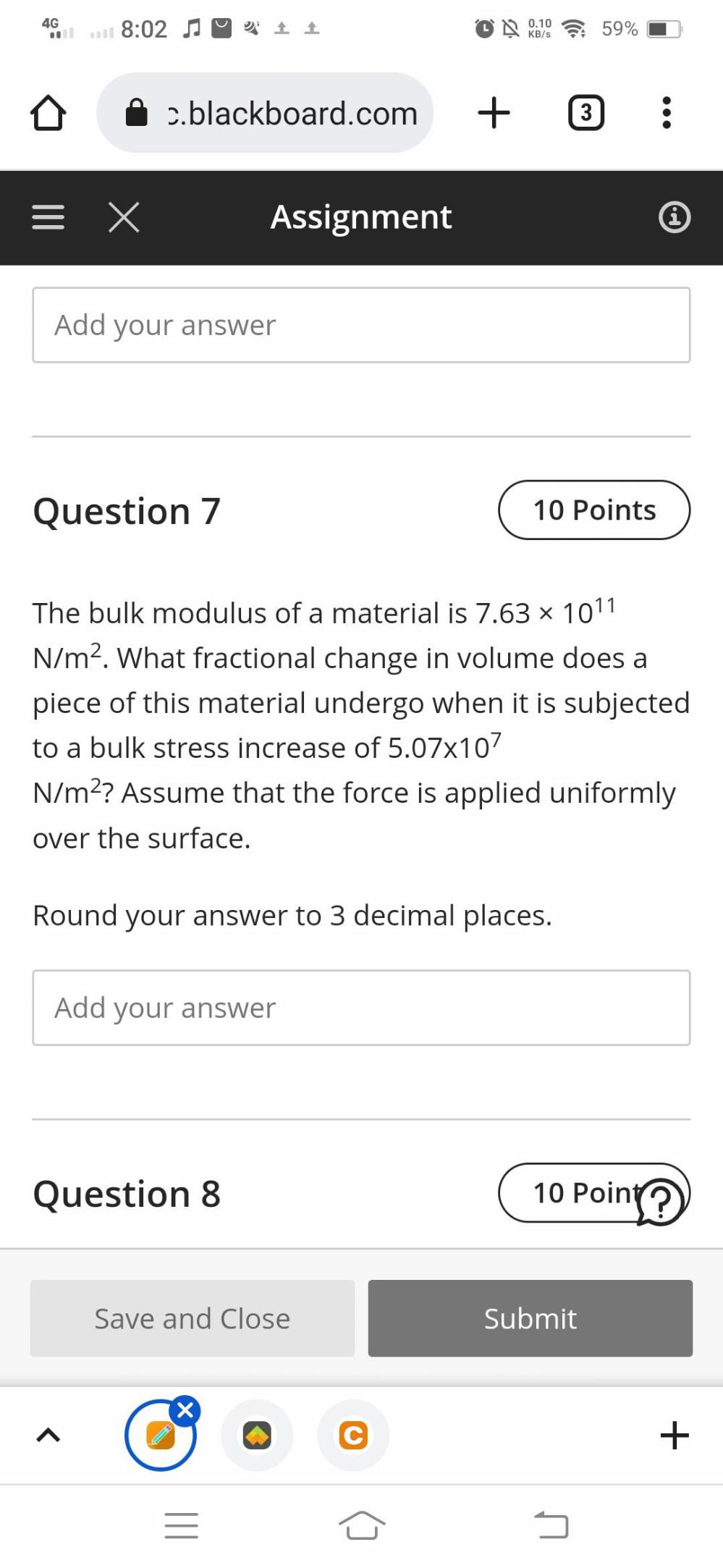Physics
Properties of matter
The bulk modulus of a material is 7.63*10¹¹ N/m². What fractional change in volume does a piece of this material undergo when it is subjected to a bulk stress increase of 5.07*10⁷ N/m²? Assume that the force is applied uniformly over the surface.